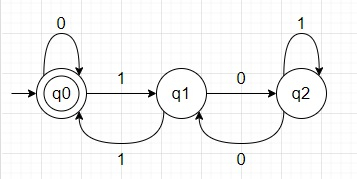# Check if binary string multiple of 3 using DFA in Python

Suppose we have an array n that represents a binary representation of any number. We have to check whether its binary representation is divisible by three or not by using Deterministic Finite Automata DFA.

So, if the input is like n = [1, 1, 0, 0] (binary of 12), then the output will be True.

To solve this, we can construct DFA like below −The approach is simple when a number is divisible by 3 then the remainder will be 0, if not then remainder will be 1 or 2. There are three states for these three remainders. The initial state is also final state because when remainder is 0 it means the number is divisible.

To solve this, we will follow these steps −

• dfa_state := 0
• for i in range 0 to size of nums - 1, do
• digit := nums[i]
• if dfa_state is 0, then
• if digit is same as 1, then
• dfa_state := 1
• otherwise when dfa_state is 1, then
• if digit is same as 0, then
• dfa_state := 2
• otherwise,
• dfa_state := 0
• otherwise when dfa_state is 2, then
• if digit is same as 0, then
• dfa_state := 1
• if dfa_state is 0, then
• return True
• return False

Let us see the following implementation to get better understanding −

## Example

Live Demo

def solve(nums):
dfa_state = 0
for i in range(len(nums)):
digit = nums[i]
if dfa_state == 0:
if digit == 1:
dfa_state = 1
elif dfa_state == 1:
if digit == 0:
dfa_state = 2
else:
dfa_state = 0
elif dfa_state == 2:
if digit == 0:
dfa_state = 1
if dfa_state == 0:
return True
return False
n = [1, 1, 0, 0]
print(solve(n))

## Input

[1, 1, 0, 0]

## Output

True